# 《莽荒纪2》公测庆典活动公告

2020-05-25 17:17:17
````【活动时间】2020/5/27 00：00：00~2020/6/2 23：59：59`

`【开放区服】新服开服1-7天或者老服时间段内开放`

`【活动规则】`

`活动一：庆典礼盒`

`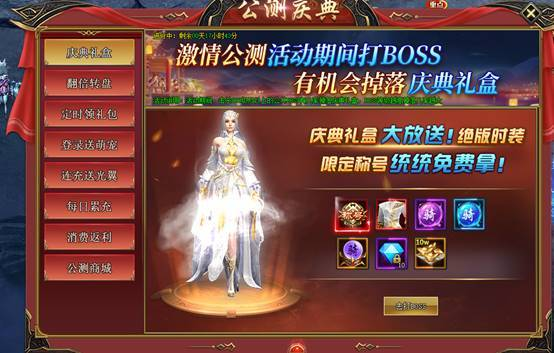`

`• 活动期间玩家击杀boss即可有概率掉落庆典礼盒，庆典礼盒中可以开出绝版时装以及绝版称号　　````
 ``道具`` ``概率`` ``坐骑进阶丹`` ``30%`` ``神装碎片`` ``5%`` ``魂之盔玄晶（中）`` ``30%`` ``5W元宝箱`` ``5%`` ``绑钻*5`` ``12%`` ``云裁玉叶时装碎片`` ``5%`` ``屠魔勇士称号`` ``3%`` ``绝学真气*100`` ``10%``
```

`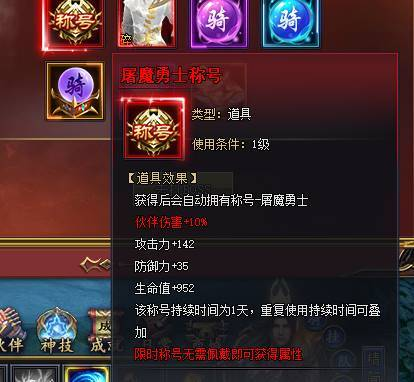`

`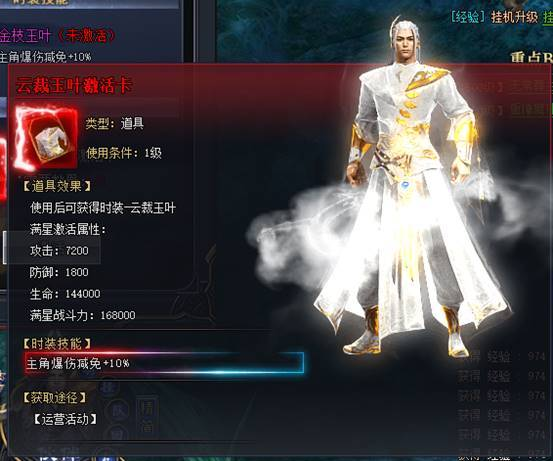`

` 活动二：钻石翻倍`

`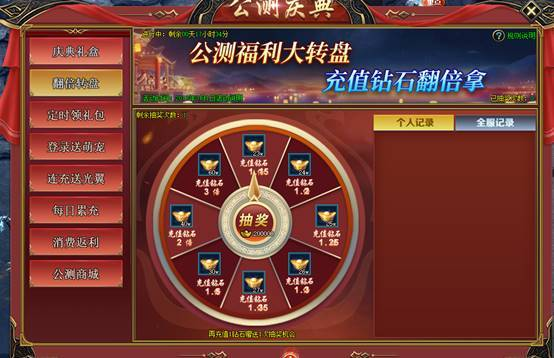`

```
 ``条件`` ``消耗`` ``可翻倍率`` ``无`` ``20W元宝`` ``1.15~3`` ``任意充值`` ``100W元宝`` ``1.15~3`` ``任意充值`` ``200绑钻`` ``1.15~3`` ``充值100钻石`` ``100钻石`` ``1.15~3`` ``充值500钻石`` ``300钻石`` ``1.15~3`` ``充值1000钻石`` ``500钻石`` ``1.15~3`` ``充值3000钻石`` ``1000钻石`` ``1.15~3`` ``充值6000钻石`` ``2000钻石`` ``1.15~3`` ``充值1W钻石`` ``4000钻石`` ``1.15~3`` ``充值18888钻石`` ``8000钻石`` ``1.15~3`` ``充值38888钻石`` ``20000钻石`` ``1.15~3`` ``充值68888钻石`` ``40000钻石`` ``1.15~3``
````说明：活动期间达成条件即可获取抽奖次数，消耗多少返还一定倍率的同类货币，今年的手气王就是你`

`活动三：定时领礼包`

`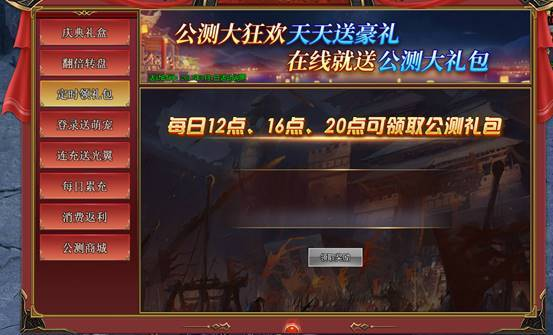`

`玩法说明：每天12点，16点，20点在线可领取庆典礼盒，每次可以领取5个；过时间点将不能领取，请及时领取奖励；`

`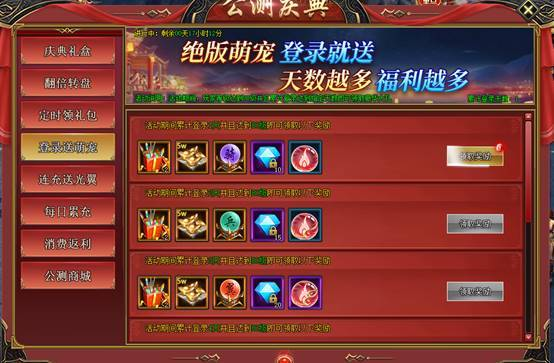`

`活动说明：活动期间坚持每日登录即可领取丰厚奖励，累计登录7天可领取一只可爱萌宠-小舞狮，附带特技-回血效果提升50%`

`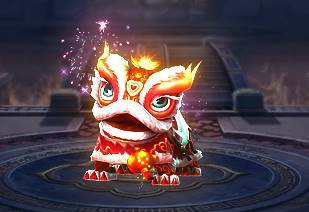````
 ``条件`` ``奖励`` ``登录第1天`` ``庆典礼盒*2`` ``5w元宝箱子*1`` ``坐骑进阶石*1`` ``绑钻*10`` ``卓越丹|1品`` ``登录第2天`` ``庆典礼盒*3`` ``5w元宝箱子*1`` ``神兵进阶石*1`` ``绑钻*15`` ``卓越丹|2品`` ``登录第3天`` ``庆典礼盒*4`` ``5w元宝箱子*1`` ``光翼进阶石*1`` ``绑钻*20`` ``卓越丹|3品`` ``登录第4天`` ``庆典礼盒*5`` ``5w元宝箱子*1`` ``剑魂进阶石*1`` ``绑钻*25`` ``卓越丹|4品`` ``登录第5天`` ``庆典礼盒*6`` ``5w元宝箱子*1`` ``仙迹进阶石*1`` ``绑钻*30`` ``特惠礼包`` ``登录第6天`` ``庆典礼盒*7`` ``5w元宝箱子*1`` ``探宝令*1`` ``绑钻*35`` ``特惠礼包`` ``登录第7天`` ``庆典礼盒*8`` ``5w元宝箱子*1`` ``探宝令*1`` ``绑钻*40`` ``特惠礼包``
``````

`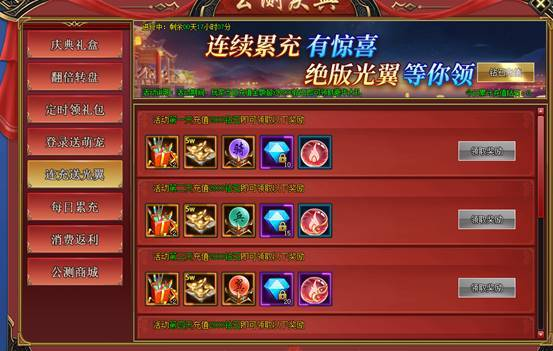`

`活动说明：活动期间每日充值满2000钻石即可领取丰厚奖励，累计充值满7天可领取炫酷光翼　　````
 ``条件`` ``奖励`` ``第1天充值满2000钻石`` ``神装碎片*20`` ``坐骑进阶丹*10`` ``50W元宝箱子*1`` ``魂之盔玄晶|中*10`` ``伙伴进阶丹*10`` ``复活丹*1`` ``第2天充值满2000钻石`` ``神装碎片*20`` ``神兵进阶丹*10`` ``50W元宝箱子*1`` ``魂之盔玄晶|中*10`` ``伙伴进阶丹*10`` ``复活丹*1`` ``第3天充值满2000钻石`` ``神装碎片*20`` ``光翼进阶丹*10`` ``50W元宝箱子*1`` ``魂之盔玄晶|中*10`` ``伙伴进阶丹*10`` ``复活丹*1`` ``第4天充值满2000钻石`` ``神装碎片*20`` ``剑魂进阶丹*10`` ``50W元宝箱子*1`` ``魂之带玄晶|中*10`` ``伙伴进阶丹*10`` ``复活丹*1`` ``第5天充值满2000钻石`` ``神装碎片*20`` ``仙迹进阶丹*10`` ``50W元宝箱子*1`` ``魂之带玄晶|中*10`` ``伙伴进阶丹*10`` ``复活丹*1`` ``第6天充值满2000钻石`` ``神装碎片*20`` ``探宝令*10`` ``50W元宝箱子*1`` ``魂之带玄晶|中*10`` ``伙伴进阶丹*10`` ``复活丹*1`` ``第7天充值满2000钻石`` ``神装碎片*20`` ``探宝令*10`` ``50W元宝箱子*1`` ``魂之戒玄晶|中*10`` ``伙伴进阶丹*10`` ``复活丹*1``
````-若昨日没有达标；可花费988钻石补签获得奖励`

```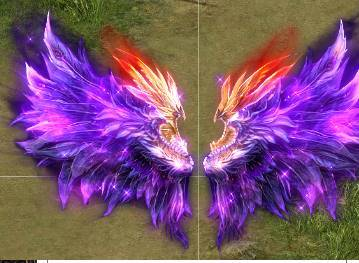-```

`活动六：每日累充`

`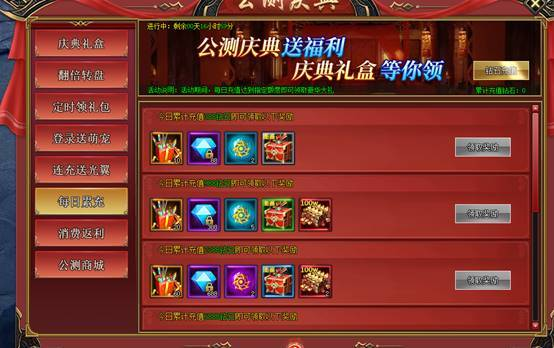````
 ``充值`` ``奖励`` ``388`` ``庆典礼盒*10`` ``少年·纪宁碎片*5`` ``伙伴进阶丹*5`` ``萌宠进阶丹*10`` ``白色图鉴随机礼盒`` ``988`` ``庆典礼盒*20`` ``少年·纪宁碎片*10`` ``伙伴进阶丹*10`` ``萌宠进阶丹*20`` ``绿色图鉴随机礼盒`` ``2388`` ``庆典礼盒*60`` ``少年·纪宁碎片*20`` ``伙伴进阶丹*15`` ``萌宠进阶丹*40`` ``蓝色图鉴随机礼盒`` ``6888`` ``庆典礼盒*120`` ``少年·纪宁碎片*40`` ``伙伴进阶丹*20`` ``萌宠进阶丹*80`` ``紫色图鉴随机礼盒`` ``15888`` ``庆典礼盒*250`` ``少年·纪宁碎片*80`` ``伙伴进阶丹*30`` ``萌宠进阶丹*150`` ``橙色图鉴随机礼盒``
````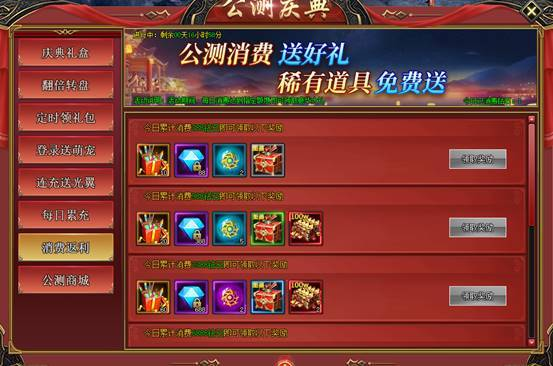`

`活动七：消费返利````
 ``消耗`` ``奖励`` ``168钻石`` `` 云裁玉叶时装碎片*1`` `` 初级伙伴属性丹*1`` `` 5w元宝箱*1`` ``388钻石`` `` 云裁玉叶时装碎片*1`` `` 初级伙伴属性丹*1`` `` 5w元宝箱*1`` ``688钻石`` `` 云裁玉叶时装碎片*1`` `` 初级伙伴属性丹*1`` `` 5w元宝箱*1`` ``1288钻石`` `` 云裁玉叶时装碎片*1`` `` 初级伙伴属性丹*1`` `` 10w元宝箱*1`` ``1888钻石`` `` 云裁玉叶时装碎片*1`` `` 初级伙伴属性丹*1`` `` 10w元宝箱*1`` ``2688钻石`` `` 云裁玉叶时装碎片*1`` `` 中级伙伴属性丹*1`` `` 10w元宝箱*1`` ``3888钻石`` `` 云裁玉叶时装碎片*1`` `` 中级伙伴属性丹*1`` `` 50w元宝箱*1`` ``5288钻石`` `` 云裁玉叶时装碎片*1`` `` 中级伙伴属性丹*1`` `` 50w元宝箱*1`` ``6888钻石`` `` 云裁玉叶时装碎片*1`` `` 中级伙伴属性丹*1`` `` 50w元宝箱*1`` ``8888钻石`` `` 云裁玉叶时装碎片*1`` `` 中级伙伴属性丹*1`` `` 50w元宝箱*1`` ``10888钻石`` `` 云裁玉叶时装碎片*1`` `` 高级伙伴属性丹*1`` `` 100w元宝箱*1`` ``13888钻石`` `` 云裁玉叶时装碎片*1`` `` 高级伙伴属性丹*1`` `` 100w元宝箱*1`` ``16888钻石`` `` 云裁玉叶时装碎片*1`` `` 高级伙伴属性丹*1`` `` 100w元宝箱*1`` ``19888钻石`` `` 云裁玉叶时装碎片*1`` `` 高级伙伴属性丹*1`` `` 100w元宝箱*1``
````活动八：公测商城`

`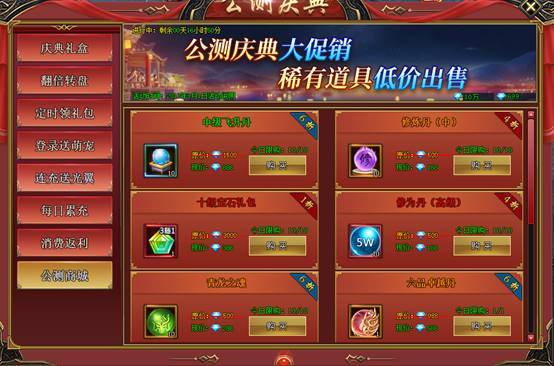````
` `
 ``开放条件`` ``物品`` ``限购次数`` ``原价`` ``价格`` ``跨服1+`` ``中级飞升丹（新道具）*10`` ``10`` ``1500`` ``888`` ``修炼丹（中）*10`` ``10`` ``500`` ``188`` ``10级宝石随机*1`` ``10`` ``3000`` ``388`` ``修为丹|5W*10`` ``10`` ``500`` ``188`` ``青龙之魂*10`` ``10`` ``500`` ``288`` ``卓越丹|6品`` ``1`` ``988`` ``588`` ``跨服8+`` ``白虎之魂*10`` ``10`` ``500`` ``288`` ``卓越丹|7品`` ``1`` ``1588`` ``588`` ``跨服15+`` ``朱雀之魂*10`` ``10`` ``500`` ``288`` ``卓越丹|8品`` ``1`` ``1988`` ``588`` ``跨服30+`` ``玄武之魂*10`` ``10`` ``500`` ``288`` ``卓越丹|9品`` ``1`` ``2588`` ``588``
``````